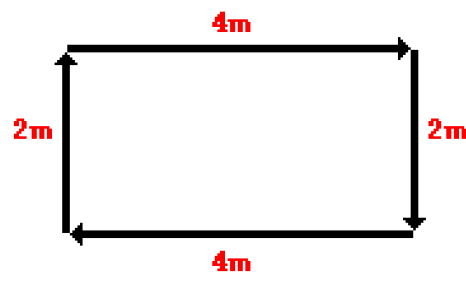# Distance and Displacement

## Introduction

Distance and displacement are two quantities that may seem to mean the same thing yet have distinctly different definitions and meanings.
• Distance is a scalar quantity that refers to "how much ground an object has covered" during its motion.
• Displacement is a vector quantity that refers to "how far out of place an object is" it is the object's overall change in position.

Let's consider the motion of a physics instructor who walked 4 meters East, 2 meters South, 4 meters West, and finally 2 meters North. See illustration below:Even though the physics instructor walked a total distance of 12 meters, his displacement is 0 meters.
Displacement, being a vector quantity, must give attention to direction.

Distance is different from Displacement:
• Distance is Scalar quantity. Displacement is Vector quantity.
• Distance is how much ground an object has covered during its motion.
• Displacement is the overall change in object position.

### Exercises: Distance and Displacement

#### Exercise 1

Adam drove off his car 1 mile North, 3 miles West, 1 mile South, and finally 3 miles km East.
Question: What is the total distance from the starting point? What is the total displacement from the starting point?